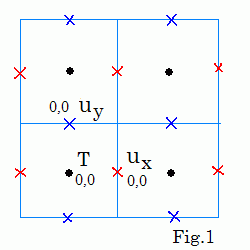It β delay fps
Fluid dynamics. Cold and heavy fluid is blue and hot fluid is red. This script makes 2 It iterations every time step to calculate pressure. 1k demo and 2k demo.

Karl Fredrickson made more accurate Fluids demo.

# 2D fluid dynamics

The Navier-Stokes equations for the fluid velocity u and the equation for temperature T of the fluid are:
t u = -(u·∇) u - (1/ρ)∇p + ν∇2u + f ,
∇·u = 0 ,
∂t T = -(u·∇)T + D∇2T + S .

where ν is the kinematic viscosity of the fluid, ρ ≡ 1 is its density, p is pressure, S is the thermal source, f = (0, -βT) is the force term, β is buoyancy.Simulation is based on the "stable fluids" method of Stam [1,2]. For simplicity the application does not perform diffusion, but the used Advection algorithm causes it to diffuse anyway (due to bilinear interpolation of the 4 closest texels). 512×512 staggered grid is used (see Fig.1). (ux , uy , T, p ) are stored in RGBA textures with periodic boundaries.
This application is simple and funny (not sure if it is accurate and efficient).

 Jos Stam   Real-Time Fluid Dynamics for Games and Stable Fluids
 Mark J. Harris   Fast Fluid Dynamics Simulation on the GPU   GPU Gems: Chapter 38

Simulations on GPU
updated 12 Aug 2016